GeeksforGeeks App
Open AppBrowser
Continue

# How To Annotate Bars in Barplot with Matplotlib in Python?

Annotation means adding notes to a diagram stating what values do it represents. It often gets tiresome for the user to read the values from the graph when the graph is scaled down or is overly populated.  In this article, we will discuss how to annotate the bar plots created in python using matplotlib library.

Following are examples of annotated and non-annotated bar plots: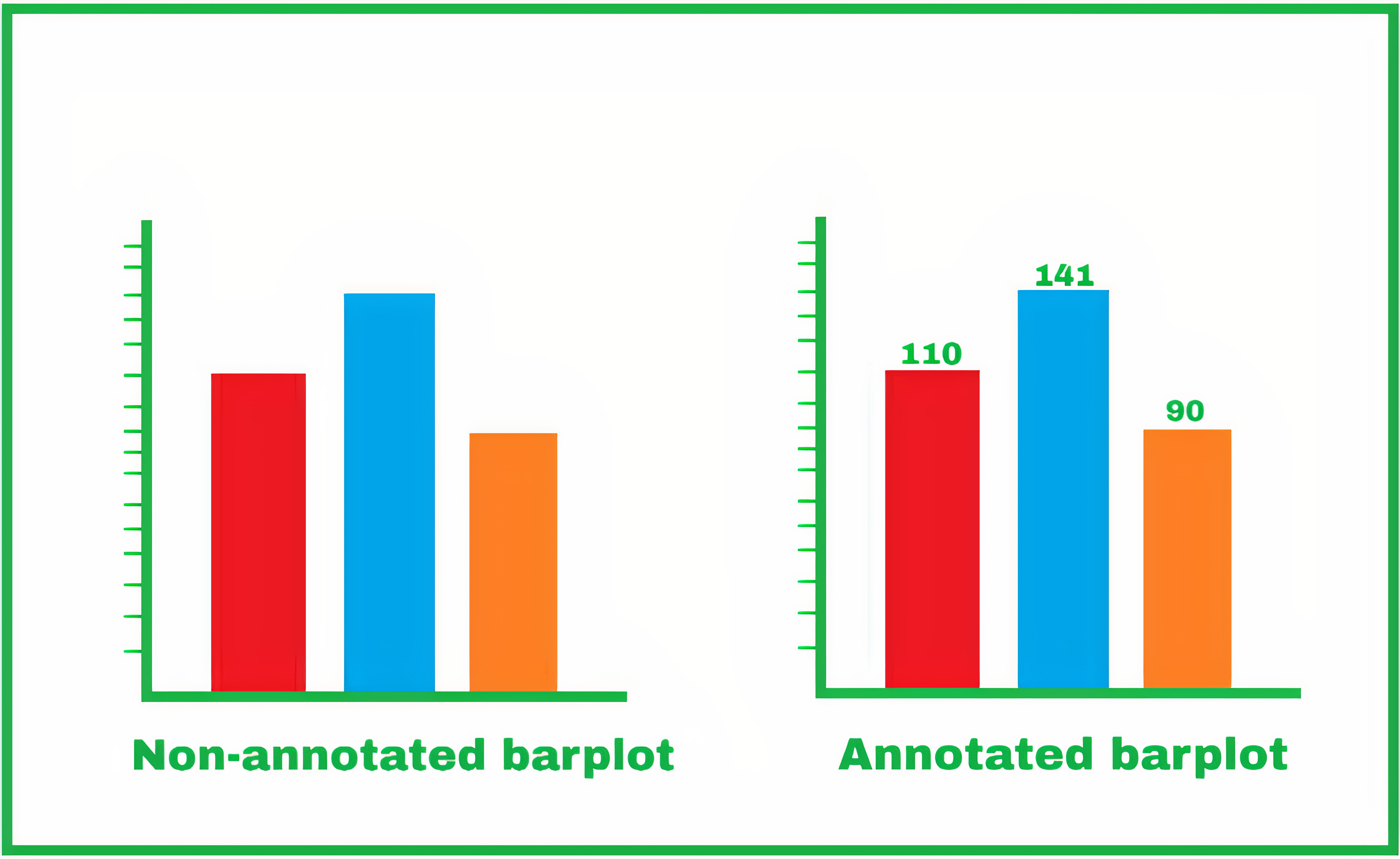Non-annotated Vs Annotated Barplot

### Step-by-step Approach:

• Let’s first plot simple graphs from a Pandas dataframe, so now we have the following dataframe ready:

## Python3

 `# Importing libraries for dataframe creation``# and graph plotting``import` `numpy as np``import` `pandas as pd``import` `seaborn as sns``import` `matplotlib.pyplot as plt` `# Creating our own dataframe``data ``=` `{``"Name"``: [``"Alex"``, ``"Bob"``, ``"Clarein"``, ``"Dexter"``],``        ``"Marks"``: [``45``, ``23``, ``78``, ``65``]}` `# Now convert this dictionary type data into a pandas dataframe``# specifying what are the column names``df ``=` `pd.DataFrame(data, columns``=``[``'Name'``, ``'Marks'``])`

Output: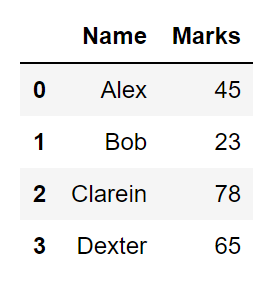User generated pandas dataframe

• Let’s now start plotting the dataframe using the seaborn library. We get the following results. But it is not quite visible that what are the actual values in the bar plots. This condition can also arise when the values of neighboring plots are quite close to each other.

## Python3

 `# Defining the plotsize``plt.figure(figsize``=``(``8``, ``6``))` `# Defining the x-axis, the y-axis and the data``# from where the values are to be taken``plots ``=` `sns.barplot(x``=``"Name"``, y``=``"Marks"``, data``=``df)` `# Setting the x-acis label and its size``plt.xlabel(``"Students"``, size``=``15``)` `# Setting the y-axis label and its size``plt.ylabel(``"Marks Secured"``, size``=``15``)` `# Finallt plotting the graph``plt.show()`

Output: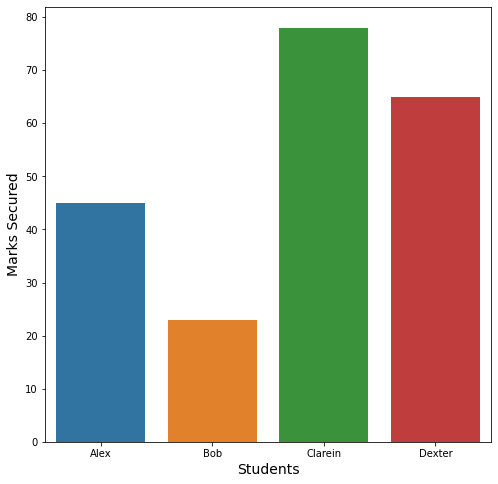Raw barplot of the dataframe

• Adding the annotations. Our strategy here will be to iterate all over the bars and put a text over all of them that will point out the values of that particular bar. Here we will use the Matlpotlib’s function called annotate(). We can find various uses of this function in various scenarios, currently, we will be just showing the value of the respective bars at their top.

Our steps will be:

1. Iterate over the bars
2. Get the x-axis position(x) and the width(w) of the bar this will help us to get the x coordinate of the text i.e. get_x()+get_width()/2.
3. The y-coordinate(y) of the text can be found using the height of the bar i.e. get_height()
4. So we have the coordinates of the annotation value i.e. get_x()+get_width()/2, get_height()
5. But this will print the annotation exactly on the boundary of the bar so to get a more pleasing annotated plot we use the parameter xyplot=(0, 8). Here 8 denotes the pixels that will be left from the top of the bar. Therefore to go below the barline we can use xy=(0,-8).
6. So we execute the following code to get the annotated graph:

## Python3

 `# Defining the plot size``plt.figure(figsize``=``(``8``, ``8``))` `# Defining the values for x-axis, y-axis``# and from which dataframe the values are to be picked``plots ``=` `sns.barplot(x``=``"Name"``, y``=``"Marks"``, data``=``df)` `# Iterating over the bars one-by-one``for` `bar ``in` `plots.patches:``  ` `  ``# Using Matplotlib's annotate function and``  ``# passing the coordinates where the annotation shall be done``  ``# x-coordinate: bar.get_x() + bar.get_width() / 2``  ``# y-coordinate: bar.get_height()``  ``# free space to be left to make graph pleasing: (0, 8)``  ``# ha and va stand for the horizontal and vertical alignment``    ``plots.annotate(``format``(bar.get_height(), ``'.2f'``),``                   ``(bar.get_x() ``+` `bar.get_width() ``/` `2``,``                    ``bar.get_height()), ha``=``'center'``, va``=``'center'``,``                   ``size``=``15``, xytext``=``(``0``, ``8``),``                   ``textcoords``=``'offset points'``)` `# Setting the label for x-axis``plt.xlabel(``"Students"``, size``=``14``)` `# Setting the label for y-axis``plt.ylabel(``"Marks Secured"``, size``=``14``)` `# Setting the title for the graph``plt.title(``"This is an annotated barplot"``)` `# Finally showing the plot``plt.show()`

Output: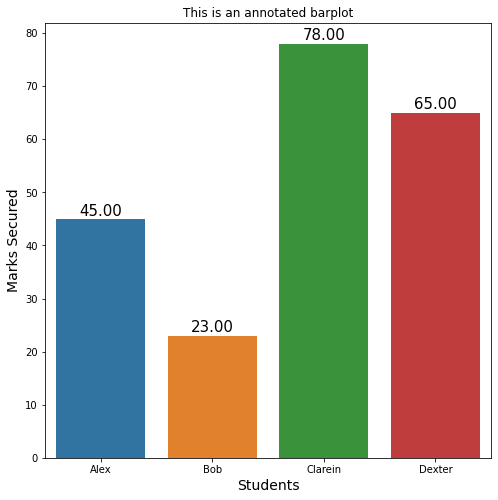Bar plot annotated with the bar values

Below is the complete program based on the above approach:

## Python3

 `# Importing libraries for dataframe creation``# and graph plotting``import` `numpy as np``import` `pandas as pd``import` `seaborn as sns``import` `matplotlib.pyplot as plt` `# Creating our own dataframe``data ``=` `{``"Name"``: [``"Alex"``, ``"Bob"``, ``"Clarein"``, ``"Dexter"``],``        ``"Marks"``: [``45``, ``23``, ``78``, ``65``]}` `# Now convert this dictionary type data into a pandas dataframe``# specifying what are the column names``df ``=` `pd.DataFrame(data, columns``=``[``'Name'``, ``'Marks'``])`  `# Defining the plot size``plt.figure(figsize``=``(``8``, ``8``))` `# Defining the values for x-axis, y-axis``# and from which dataframe the values are to be picked``plots ``=` `sns.barplot(x``=``"Name"``, y``=``"Marks"``, data``=``df)` `# Iterating over the bars one-by-one``for` `bar ``in` `plots.patches:``  ` `  ``# Using Matplotlib's annotate function and``  ``# passing the coordinates where the annotation shall be done``  ``# x-coordinate: bar.get_x() + bar.get_width() / 2``  ``# y-coordinate: bar.get_height()``  ``# free space to be left to make graph pleasing: (0, 8)``  ``# ha and va stand for the horizontal and vertical alignment``  ``plots.annotate(``format``(bar.get_height(), ``'.2f'``),``                   ``(bar.get_x() ``+` `bar.get_width() ``/` `2``,``                    ``bar.get_height()), ha``=``'center'``, va``=``'center'``,``                   ``size``=``15``, xytext``=``(``0``, ``8``),``                   ``textcoords``=``'offset points'``)` `# Setting the label for x-axis``plt.xlabel(``"Students"``, size``=``14``)` `# Setting the label for y-axis``plt.ylabel(``"Marks Secured"``, size``=``14``)` `# Setting the title for the graph``plt.title(``"This is an annotated barplot"``)` `# Finally showing the plot``plt.show()`

Output:Bar plot annotated with the bar values

Example 1:

## Python3

 `# Importing libraries for dataframe creation``# and graph plotting``import` `numpy as np``import` `pandas as pd``import` `seaborn as sns``import` `matplotlib.pyplot as plt` `# Creating our own dataframe``data ``=` `{``"Language"``: [``"Python"``, ``"C++"``, ``"Java"``],``        ``"Students"``: [``75``, ``50``, ``25``]}` `# Now convert this dictionary type data into a pandas dataframe``# specifying what are the column names``df ``=` `pd.DataFrame(data, columns``=``[``'Language'``, ``'Students'``])`  `# Defining the plot size``plt.figure(figsize``=``(``5``, ``5``))` `# Defining the values for x-axis, y-axis``# and from which dataframe the values are to be picked``plots ``=` `sns.barplot(x``=``"Language"``, y``=``"Students"``, data``=``df)` `# Iterating over the bars one-by-one``for` `bar ``in` `plots.patches:``  ` `    ``# Using Matplotlib's annotate function and``    ``# passing the coordinates where the annotation shall be done``    ``plots.annotate(``format``(bar.get_height(), ``'.2f'``),``                   ``(bar.get_x() ``+` `bar.get_width() ``/` `2``,``                    ``bar.get_height()), ha``=``'center'``, va``=``'center'``,``                   ``size``=``15``, xytext``=``(``0``, ``5``),``                   ``textcoords``=``'offset points'``)`  `# Setting the title for the graph``plt.title(``"Example 1"``)` `# Finally showing the plot``plt.show()`

Output: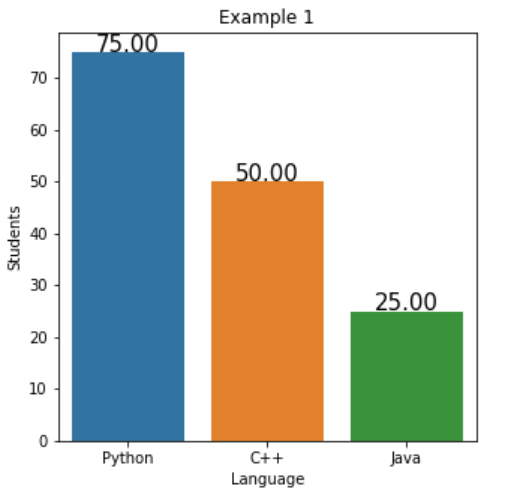Example 2:

## Python3

 `# Importing libraries for dataframe creation``# and graph plotting``import` `numpy as np``import` `pandas as pd``import` `seaborn as sns``import` `matplotlib.pyplot as plt` `# Creating our own dataframe``data ``=` `{``"Section"``: [``"A"``, ``"B"``, ``"C"``, ``'D'``, ``'E'``],``        ``"Students"``: [``0``, ``10``, ``20``, ``30``, ``40``]}` `# Now convert this dictionary type data into a pandas dataframe``# specifying what are the column names``df ``=` `pd.DataFrame(data, columns``=``[``'Section'``, ``'Students'``])`  `# Defining the plot size``plt.figure(figsize``=``(``5``, ``5``))` `# Defining the values for x-axis, y-axis``# and from which dataframe the values are to be picked``plots ``=` `sns.barplot(x``=``"Section"``, y``=``"Students"``, data``=``df)` `# Iterating over the bars one-by-one``for` `bar ``in` `plots.patches:` `    ``# Using Matplotlib's annotate function and``    ``# passing the coordinates where the annotation shall be done``    ``plots.annotate(``format``(bar.get_height(), ``'.2f'``),``                   ``(bar.get_x() ``+` `bar.get_width() ``/` `2``,``                    ``bar.get_height()), ha``=``'center'``, va``=``'center'``,``                   ``size``=``15``, xytext``=``(``0``, ``5``),``                   ``textcoords``=``'offset points'``)`  `# Setting the title for the graph``plt.title(``"Example 2"``)` `# Finally showing the plot``plt.show()`

## Python3

 `# Importing libraries for dataframe creation``# and graph plotting``import` `numpy as np``import` `pandas as pd``import` `seaborn as sns``import` `matplotlib.pyplot as plt` `# Creating our own dataframe``data ``=` `{``"Section"``: [``"A"``, ``"B"``, ``"C"``, ``'D'``, ``'E'``],``        ``"Students"``: [``0``, ``10``, ``20``, ``30``, ``40``]}` `# Now convert this dictionary type data into a pandas dataframe``# specifying what are the column names``df ``=` `pd.DataFrame(data, columns``=``[``'Section'``, ``'Students'``])`  `# Defining the plot size``plt.figure(figsize``=``(``5``, ``5``))` `# Defining the values for x-axis, y-axis``# and from which dataframe the values are to be picked``plots ``=` `sns.barplot(x``=``"Section"``, y``=``"Students"``, data``=``df)` `# Iterating over the bars one-by-one``for` `bar ``in` `plots.patches:` `    ``# Using Matplotlib's annotate function and``    ``# passing the coordinates where the annotation shall be done``    ``plots.annotate(``format``(bar.get_height(), ``'.2f'``),``                   ``(bar.get_x() ``+` `bar.get_width() ``/` `2``,``                    ``bar.get_height()), ha``=``'center'``, va``=``'center'``,``                   ``size``=``15``, xytext``=``(``0``, ``5``),``                   ``textcoords``=``'offset points'``)`  `# Setting the title for the graph``plt.title(``"Example 2"``)` `# Finally showing the plot``plt.show()`

Output: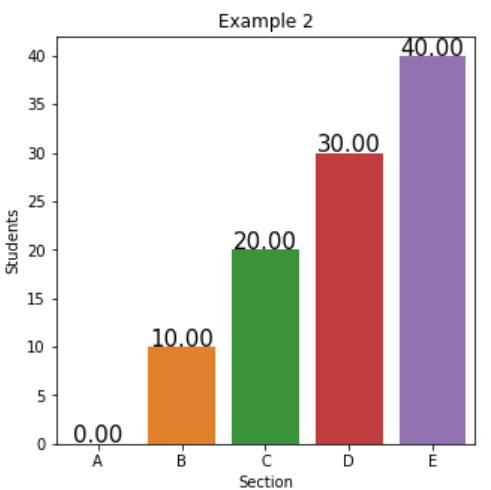My Personal Notes arrow_drop_up